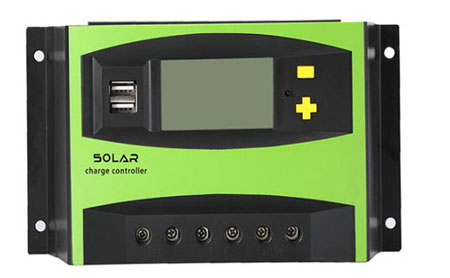# What is PWM Charging Mode of Solar Charge Controller?

The early photovoltaic controllers were all PWM. The electrical structure was simple. The controller consisted of a main power switch, a capacitor, and a drive and protection circuit. The output voltage was controlled by the PWM duty cycle of the switch tube.

PWM controller, there is only one switch between the solar array and the battery panel. As the battery is gradually filled and the battery voltage rises, the PWM controller will gradually reduce the power provided to the battery. The photovoltaic output will be output according to the maximum power. PWM controller, with battery charging and discharging management function, can prevent the battery from overcharging and over-discharging.

Since there is only one switch connected between the solar module and the storage battery of the PWM type controller, and there is no inductive voltage divider in the middle, the voltage of the module is about 1.2-2.0 times the voltage of the battery when designing, such as a 24V battery, the input of the component The voltage is between 30-50V, and each string can only be equipped with one module. For a 48V battery, the input voltage of the module is between 60-80V, and each string can only be equipped with two modules.The solar controller can also be said to be a solar charge controller. It is a controller with two charging modes, one is MPPT charging mode, and the other is PWM charging mode. Now Inverter.com will explain to you the PWM charging mode.

The PWM controller is pulse width modulated (PWM), which refers to the use of the digital output of the microprocessor to control the analog circuit, which is a way of digitally encoding the analog signal level. Then control the analog circuit in a digital way, which can greatly reduce the cost and power consumption of the system. Many microcontrollers now have PWM controllers in them.

## Principle of PWM Controller

The principle of the PWM controller is based on the fact that narrow pulses with equal impulse but different shapes have the same effect when applied to the link with inertia. PWM control principle: divide the waveform into 6 equal parts, which can be equivalently replaced by these 6 square waves. There are many classification methods of pulse width modulation, such as single polarity and bipolarity, synchronous and asynchronous, rectangular wave modulation and sine wave modulation, and so on.

Unipolar PWM control means that the carrier only changes in one direction within half a period, and the resulting PWM waveform only changes in one direction. The bipolar PWM control method is the opposite of the unipolar one, which changes the carrier in two directions within half a cycle. According to whether the carrier signal is synchronized with the modulation signal, PWM control can be divided into synchronous modulation and asynchronous modulation. The characteristic of rectangular wave pulse width modulation is that the output pulse width columns are of equal width, and can only control harmonics of a certain order; The output waveform is close to a sine wave. Sine wave pulse width modulation is also called SPWM. Generating pulse width according to the control signal is the key to this technology. At present, the triangular wave comparison method, the hysteresis comparison method, and the space voltage vector method are commonly used.

Buy a high efficiency solar charge controller from Inverter.com, including 10 Amp20 Amp,...60 Amp PWM solar charge controllers and 20A30A to 60A MPPT solar charge controllers.